GATE  >  Test: Geotechnical Engineering- 1

# Test: Geotechnical Engineering- 1

Test Description

## 15 Questions MCQ Test GATE Civil Engineering (CE) 2023 Mock Test Series | Test: Geotechnical Engineering- 1

Test: Geotechnical Engineering- 1 for GATE 2023 is part of GATE Civil Engineering (CE) 2023 Mock Test Series preparation. The Test: Geotechnical Engineering- 1 questions and answers have been prepared according to the GATE exam syllabus.The Test: Geotechnical Engineering- 1 MCQs are made for GATE 2023 Exam. Find important definitions, questions, notes, meanings, examples, exercises, MCQs and online tests for Test: Geotechnical Engineering- 1 below.
Solutions of Test: Geotechnical Engineering- 1 questions in English are available as part of our GATE Civil Engineering (CE) 2023 Mock Test Series for GATE & Test: Geotechnical Engineering- 1 solutions in Hindi for GATE Civil Engineering (CE) 2023 Mock Test Series course. Download more important topics, notes, lectures and mock test series for GATE Exam by signing up for free. Attempt Test: Geotechnical Engineering- 1 | 15 questions in 45 minutes | Mock test for GATE preparation | Free important questions MCQ to study GATE Civil Engineering (CE) 2023 Mock Test Series for GATE Exam | Download free PDF with solutions
 1 Crore+ students have signed up on EduRev. Have you?
Test: Geotechnical Engineering- 1 - Question 1

### Which one of the following relations is not correct?

Detailed Solution for Test: Geotechnical Engineering- 1 - Question 1

The correct relations are as follows:
e=n/(1-n)
γs=((G+e)/(1+e)) γw
n=e/(1+e)
e=wG/S

Test: Geotechnical Engineering- 1 - Question 2

### The void ratio-pressure diagram is shown in figure given below. The coefficient of compressibility is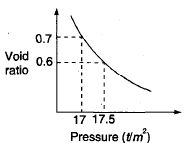Detailed Solution for Test: Geotechnical Engineering- 1 - Question 2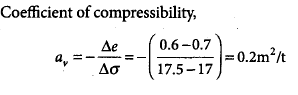Test: Geotechnical Engineering- 1 - Question 3

### Standard Newmark’s influence chart is shown in the given figure. if loaded equally, the areas marked 1 and 2 will yield pressures at the center such that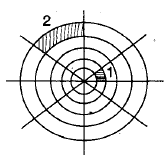Detailed Solution for Test: Geotechnical Engineering- 1 - Question 3

Any subarea of Newmark’s chart has same influence value. Therefore, 1 and 2 will yield same pressure at the center.

Test: Geotechnical Engineering- 1 - Question 4

A saturates soil mass has a total density 22 kN/m3 and a water content of 10%. The bulk density and dry density of this soil are

Detailed Solution for Test: Geotechnical Engineering- 1 - Question 4

For saturated soil,

γbulk = γsat = 22 kN/m3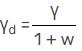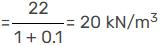Test: Geotechnical Engineering- 1 - Question 5

The factor of safety of a slope of infinite extent having a slope angle = 25°, and the slope is made of cohensionless soil with φ = 30° is.

Detailed Solution for Test: Geotechnical Engineering- 1 - Question 5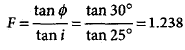Test: Geotechnical Engineering- 1 - Question 6

In a three-layered soil, water flows parallel to stratification. The thickness of the middle layer is twice that of the top and bottom layer. The coefficient of permeability of middle layer (2K) is twice that of top and bottom layer (K). What is the average coefficient of permeability for this flow?

Detailed Solution for Test: Geotechnical Engineering- 1 - Question 6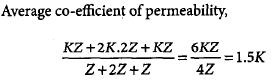Test: Geotechnical Engineering- 1 - Question 7

The dry density of a soil is 1.5 g/cc. if the saturation water content is 50%, then its saturated density and submerged density would, respectively, be

Detailed Solution for Test: Geotechnical Engineering- 1 - Question 7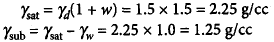Test: Geotechnical Engineering- 1 - Question 8

A soil has a bulk density of 1.80 g/cm3 at water content of 5%. If the void ratio remains constant, then its bulk density for the water content of 10% will be

Detailed Solution for Test: Geotechnical Engineering- 1 - Question 8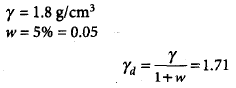Voids ratio does not change after drying. Therefore, γd remains same.
γ = γd(1 + w) = 1.71(1 + 0.1) = 1.88 g/cm2

Test: Geotechnical Engineering- 1 - Question 9

____________ is the measure of loss of strength with remoulding, with water content unchanged.

Detailed Solution for Test: Geotechnical Engineering- 1 - Question 9

Sensitivity values ranges from 1-16. For an insensitive soil, it is 1. A soil with sensitivity above 16, is termed as quick.

Test: Geotechnical Engineering- 1 - Question 10

In Geotechnical Engineering, soil is considered as a _________ phase material.

Detailed Solution for Test: Geotechnical Engineering- 1 - Question 10

Soil is a 3 phase material. It consists of solids, water and air voids. It is represented in a 3 phase diagram with volume on left side and mass on the right side.

*Answer can only contain numeric values
Test: Geotechnical Engineering- 1 - Question 11

In a plate load test on sandy soil, the test plate of 60 cm × 60 cm undergoes a settlement of 5 mm at a pressure of 12 × 104 N/m2. The expected settlement of 3 m × 3 m footing under same pressure will be _________ mm.

Detailed Solution for Test: Geotechnical Engineering- 1 - Question 11

Sf = Sp* { Bf (Bp + .3)/Bp (Bf+ .3) }2
= 5 * { 3 ( .6+ .3)/ .6 ( 3+ .3)}2
= 9.24 mm.

Test: Geotechnical Engineering- 1 - Question 12

A normally consolidated clay layer settles by 25 mm when the effective stress is increased from 15 to 30 kPa. If the effective stress is later increased further from 30 kPa to 60 kPa, then the additional settlement would be

Detailed Solution for Test: Geotechnical Engineering- 1 - Question 12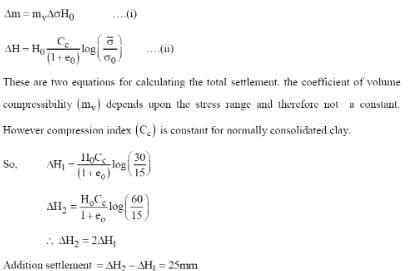Test: Geotechnical Engineering- 1 - Question 13

A flownet constructed to determine the seepage through an earth dam which is homogenous but anisotropic, gave four flow channels and sixteen equipotential drops. The coefficients of permeability in the horizontal and vertical directions are 4 × 10–7 m/s and 1.0 × 10–7 m/s, respectively. If the storage head was 20 m, then the seepage per unit length of the dam (in m3/s) would be

Detailed Solution for Test: Geotechnical Engineering- 1 - Question 13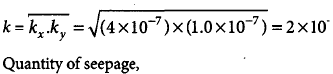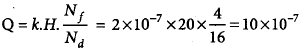*Answer can only contain numeric values
Test: Geotechnical Engineering- 1 - Question 14

A clay soil specimen tested in unconfined condition gave an unconfined compressive strength of 100 kN/m2. A specimen of the same clay with the same initial condition is subjected to a UU triaxial test under a cell pressure of 100 kN/m2. The axial stress (in kN/m2) at failure would be ____________.

Detailed Solution for Test: Geotechnical Engineering- 1 - Question 14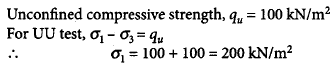Test: Geotechnical Engineering- 1 - Question 15

The initial and final void ratios of a clay sample in a consolidation  test are 1 and 0.5, respectively. If the initial thickness of the sample is 2.4 cm, then its final thickness will be

Detailed Solution for Test: Geotechnical Engineering- 1 - Question 15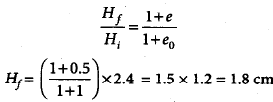## GATE Civil Engineering (CE) 2023 Mock Test Series

26 docs|292 tests
 Use Code STAYHOME200 and get INR 200 additional OFF Use Coupon Code
Information about Test: Geotechnical Engineering- 1 Page
In this test you can find the Exam questions for Test: Geotechnical Engineering- 1 solved & explained in the simplest way possible. Besides giving Questions and answers for Test: Geotechnical Engineering- 1, EduRev gives you an ample number of Online tests for practice

## GATE Civil Engineering (CE) 2023 Mock Test Series

26 docs|292 tests